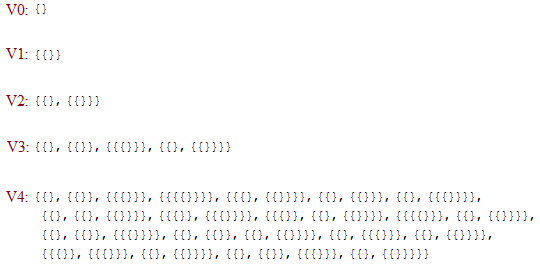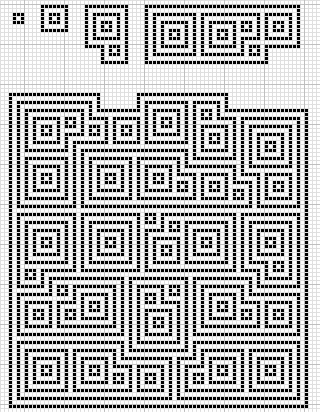# Von Neumann universe

The von Neumann universe V is the hierarchy of all set-theoretic sets. It is itself too large to be a set, and therefore is considered to be a proper class. There’s a very simple systematic construction of the von Neumann universe, beginning with the empty set, V0.

• V0 = Ø = {}

We then obtain the next stage by taking the power set of the previous stage. They get very large very quickly, but the next four stages are easily manageable. V1 is the set containing the empty set, and V2 is the set containing both the empty set and the set containing the empty set. V0 to V4, inclusive, are given below:V5 is the power set of V4, and is too large to embed into this post. Nevertheless, I’ve made a zipped text file (206 kB; decompresses to 8960 kB) containing V5 in all its glory. So, it’s small enough to store as a reasonably sized text file, and when compressed it even fits on a 3½-inch floppy.

V6 is a different story altogether. Whereas the observable universe contains about 10^79 atoms, V6 contains approximately$2.00 \times 10^{19728}$ sets. So it’s really big. In general, Vn contains$2 \uparrow \uparrow n$ sets.

Continuing in this way, we get Vn for all positive integers n. Taking their union gives the countably infinite set Vω, which contains all sets expressible as finite strings of curly brackets and commas. More generally, if α is a limit ordinal, then we define Vα to be the union of Vβ for all β < α.

Now that we have the successor and arbitrary limit operations, we can reach arbitrary ordinals in this manner.

Taking the union over all Vα gives V, the von Neumann universe, or ‘collection of all sets’.

### Packing problems

Instead of drawing curly brackets and commas, we can represent sets as closed curves, with their elements drawn within. Insisting that we draw them on a grid, with walls and gaps of width 1 (Chebyshev distance), here are diagrams of V0 to V4:The representations of V0 to V3 are definitely optimal. On the other hand, I was somewhat disappointed that I couldn’t quite get V4 to fit in a 75 by 75 square (the overhang increases it to 75 by 79). This suggests a few exercises to the reader:

• Can we fit V4 in a 75 × 75 square?
• More generally, for which a, b (of the form 4k + 3, naturally) can we fit V4 in an a × b box? The minimal width is 19.
• What is the width of the smallest square that can accommodate V5? A very weak upper bound is 20483, and I’m sure that you can do much better (below 15000, probably).
• Does anyone want to create an image of V5?
This entry was posted in Uncategorized. Bookmark the permalink.

### 8 Responses to Von Neumann universe

1.wojowu says:

You were like “I have found a truly marvelous packing of this set that 75×75 box is too narrow to contain.”

2.wojowu says:

You were like “I have found a truly marvelous packing of this set that 75×75 box was too narrow to contain.”

3.Wikipedia says:

They likely will verify nothing but the documents you extend them.
He should certainly then deposit your investments directly interested in that savings account. http://www.wikipedia.org

4.Daniel Ketterer says:

I believe it should be 2 ^ ^ ( n – 1 )
V0 is 0 and doesn’t fit, but it’s the empty set so..
V1 is 1
V2 is 2 = 2 ^ ^ 1
V3 is 4 = 2 ^ ^ 2
V4 is 16 = 2 ^ ^ 3
V5 is = 2 ^ ^ 4 = 16